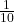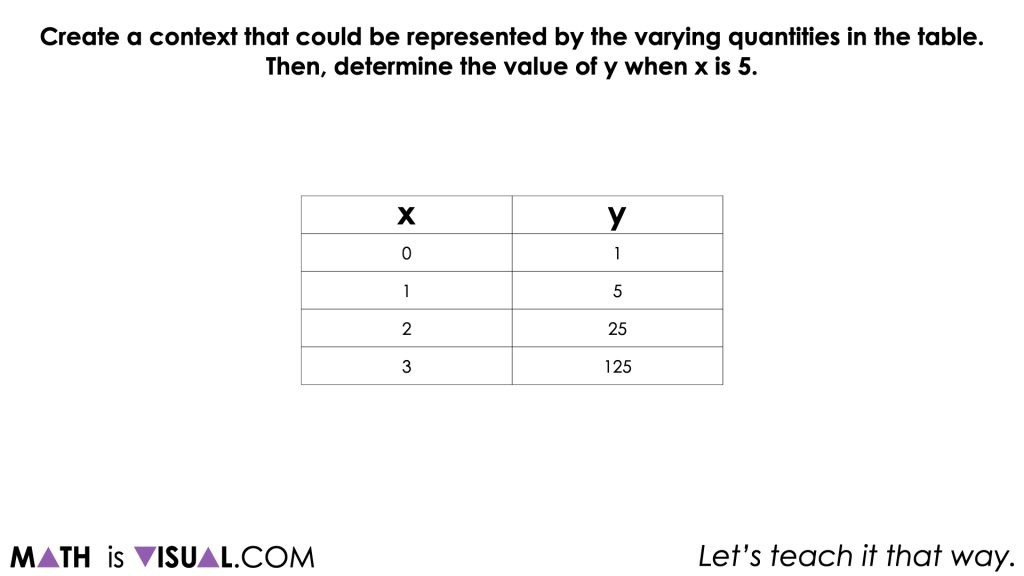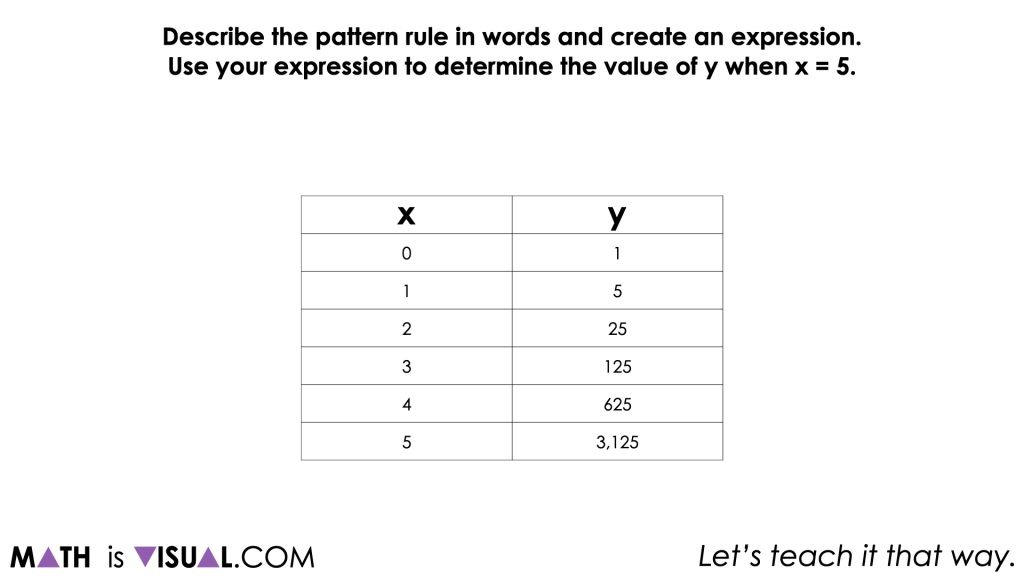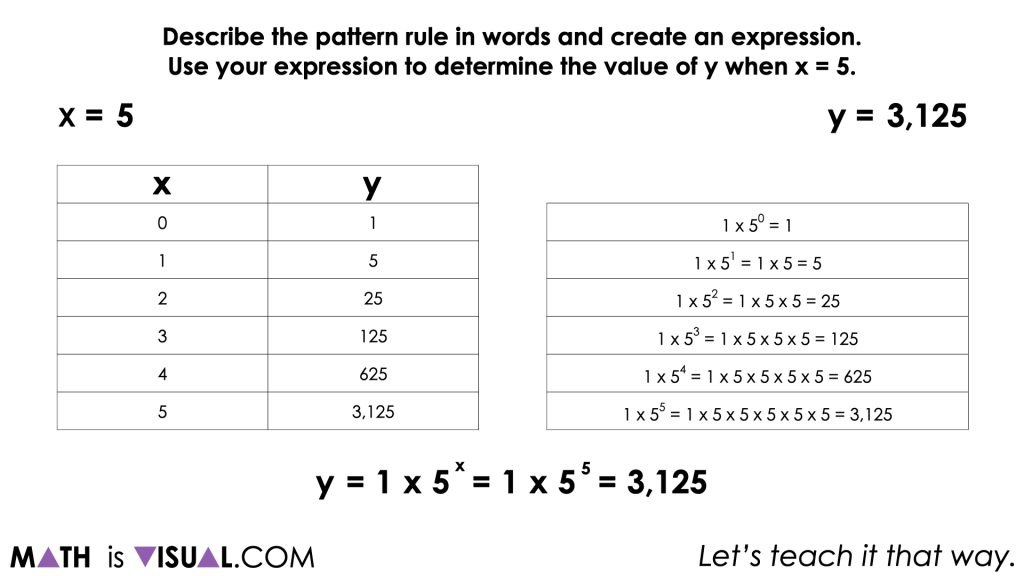# More Exponential Growth Patterns

Investigate subtracting negative numbers by finding the difference in temperature using the thermometer as a linear model number line.

## In This Set of Visual Number Talk Prompts…

Students will extend patterns with exponential growth and decay, make near and far predictions about the pattern, describe the pattern in words, and determine the general term.

## String of Related Problems

Share the following tables one at a time. Ask students to share what they know about the pattern. Develop a context that could be represented by this pattern. Describe the pattern rule in words. Collaboratively create an expression for each pattern by writing an expression. Use the expression to determine the value of the 5th term.

The string of exponential growth and decay patterns include:

y = 5x
y = 8000 () x
y = 729 () x

## Visual Math Talk Prompt #1

Students will be prompted with:

Create a context that could be represented by the varying quantities in the table.
Then, determine the value of y when x is 5.Students may attempt to extend the table and identify the pattern relating the dependent variable, y, to the independent variable, x. In this case, as x increases by 1, y increases by a factor of 5.

Are students able to articulate how y changes relative to x?

Ask students how they might identify whether the pattern is increasing linearly or not.

What might we look for to determine whether y increases additively or multiplicatively?

The contexts students create may be related to plant growth since that was the context we shared this unit, while others might extend to human or animal population growth, disease, or even a “chain email” where people have to forward an email to 5 people, then they all have to forward the email to 5 more people, etc.

Prompt students with:

Describe the pattern rule in words and create an expression.
Use your expression to determine the value of y when x = 5.While some students may struggle to describe the pattern rule in words and in particular with creating an expression, use this opportunity to explicitly highlight the verbal and algebraic pattern rules (i.e.: the expression and/or equation) to ensure they understand how this relationship is increasing.## Want to Explore These Concepts & Skills Further?

Two (2) additional number talk prompts are available in Day 4 of the Invasive Species problem based math unit that you can dive into now.

Why not start from the beginning of this contextual 5-day unit of real world lessons from the Make Math Moments Problem Based Units page.

Did you use this in your classroom or at home? How’d it go? Post in the comments!

Math IS Visual. Let’s teach it that way.